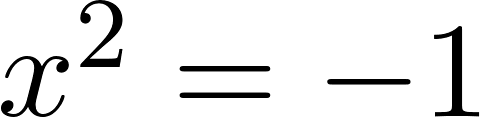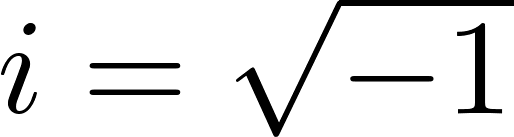### 1.1 Radicals and Complex Numbers

 Simplifying radical expressions and solving radical equations were a focus of your previous algebra course. However, your solutions to these problems dealt with only a specific type of numbers: REAL NUMBERS.Besides zero, anytime you multiply a real number by itself, you get a positive number as the answer.Solving the equationshould look something like the following:Technically, it is not possible to solve for a square root of a negative number unless you use your imagination. Because only real numbers can be multiplied by themselves to yield a positive number, mathematicians created a new type of numbers. The opposite of “real” is “not real” so they are called IMAGINARY NUMBERS.Key Termsradicalscomplex numbersimaginary numbersEssential QuestionsHow do the arithmetic operations on numbers extend to complex numbers?Practice ProblemsExtra Practice Problems - Solving Radical Equations